# MCQs on Electric Circuits

##### Page 1 of 63. Go to page 1 2 3 4 5 6 7 8 9 10 11 12 13 14 15 16 17 18 19 20 21 22 23 24 25 26 27 28 29 30 31 32 33 34 35 36 37 38 39 40 41 42 43 44 45 46 47 48 49 50 51 52 53 54 55 56 57 58 59 60 61 62 63
01․ If 1 A current flows in a circuit, the number of electrons flowing through this circuit is
0.625 × 1019
1.6 × 1019
1.6 × 10 - 19
0.625 × 10 - 19

The charge of one electron is 1.6 × 10 - 19 coulomb. Again 1 A current means transferring of 1 coulomb charge per one second.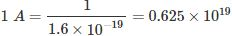02․ The resistivity of the conductor depends on
area of the conductor.
length of the conductor.
type of material.
none of these.

The resistivity is a property of a material, defined as the resistance between two opposite faces of a cube of a material of unit volume. That is why resistivity is only the unique property of a material and it does not depend upon the dimension of any piece of material. The same can also be explained as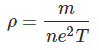Where, ρ is the resistivity; m and e is the mass and charge of an electron; T is the relaxation time. All these are properties of a material and independent of length and cross sectional area.

03․ The resistance of a conductor of diameter d and length l is R Ω. If the diameter of the conductor is halved and its length is doubled, the resistance will be
R Ω
2R Ω
4R Ω
8R Ω

As per law of resistance, the resistance of the conductor is inversely proportional to its cross-sectional area i.e. it is inversely proportional to the square of the diameter of cross-sectional area of the conductor. As per same law of resistance, the resistance of the conductor is directly proportional to the length of the conductor.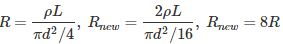04․ How many coulombs of charge flow through a circuit carrying a current of 10 A in 1 minute?
10
60
600
1200

1 Ampere current means flowing of 1 Coulomb charge per second. That means 10 A current in 1 minute or 60 seconds implies 10 × 60 = 600 coulombs.

05․ A capacitor carries a charge of 0.1 C at 5 V. Its capacitance is
0.02 F
0.5 F
0.05 F
0.2 F

The capacitance of a capacitor is expressed by Q/V. Where Q is the charge of the capacitor and V is the voltage across the capacitor.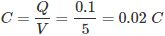06․ To obtain a high value of capacitance, the permittivity of dielectric medium should be
low
zero
high
unity

The expression of capacitance is given as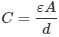Where ε is the permittivity of the medium. Hence it is seen that the capacitance of a capacitor is directly proportional to the permittivity of the medium used as dielectric. Therefore to obtain a high value of capacitance, the permittivity of dielectric medium should be high.

07․ Four capacitors each of 40 µF are connected in parallel, the equivalent capacitance of the system will be
160 µF
10 µF
40 µF
5 µF

The impedance of a capacitor is inversely proportional to its capacitance value. Reciprocal of equivalent impedance of parallel connected circuit elements is sum of reciprocal of impedance of each of the elements.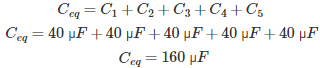08․ Five capacitors each of 5 µF are connected in series, the equivalent capacitance of the system will be
5 µF
25 µF
10 µF
1 µF

When numbers of circuit elements are connected in series, the impedance of equivalent combination is sum of impedance of all elements in series. Again, capacitance is inversely proportional to impedance. Hence, when capacitors are connected in series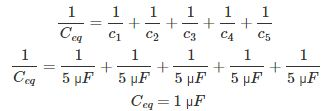09․ 1 F is theoretically equal to
1 ohm of resistance
ratio of 1 V to 1 C
ratio of 1 C to 1 V
none of these

1 Farad capacitance is defined as the capacity of dielectric medium to store 1 Coulomb charge when 1 Volt potential difference is applied across it. Thus, capacitance is expressed as the ratio of charge to voltage (Q = CV).

10․ The unit of resistivity is
Ω.
Ω - metre.
Ω / metre.
Ω / m².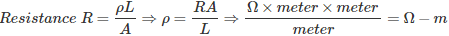123>>>